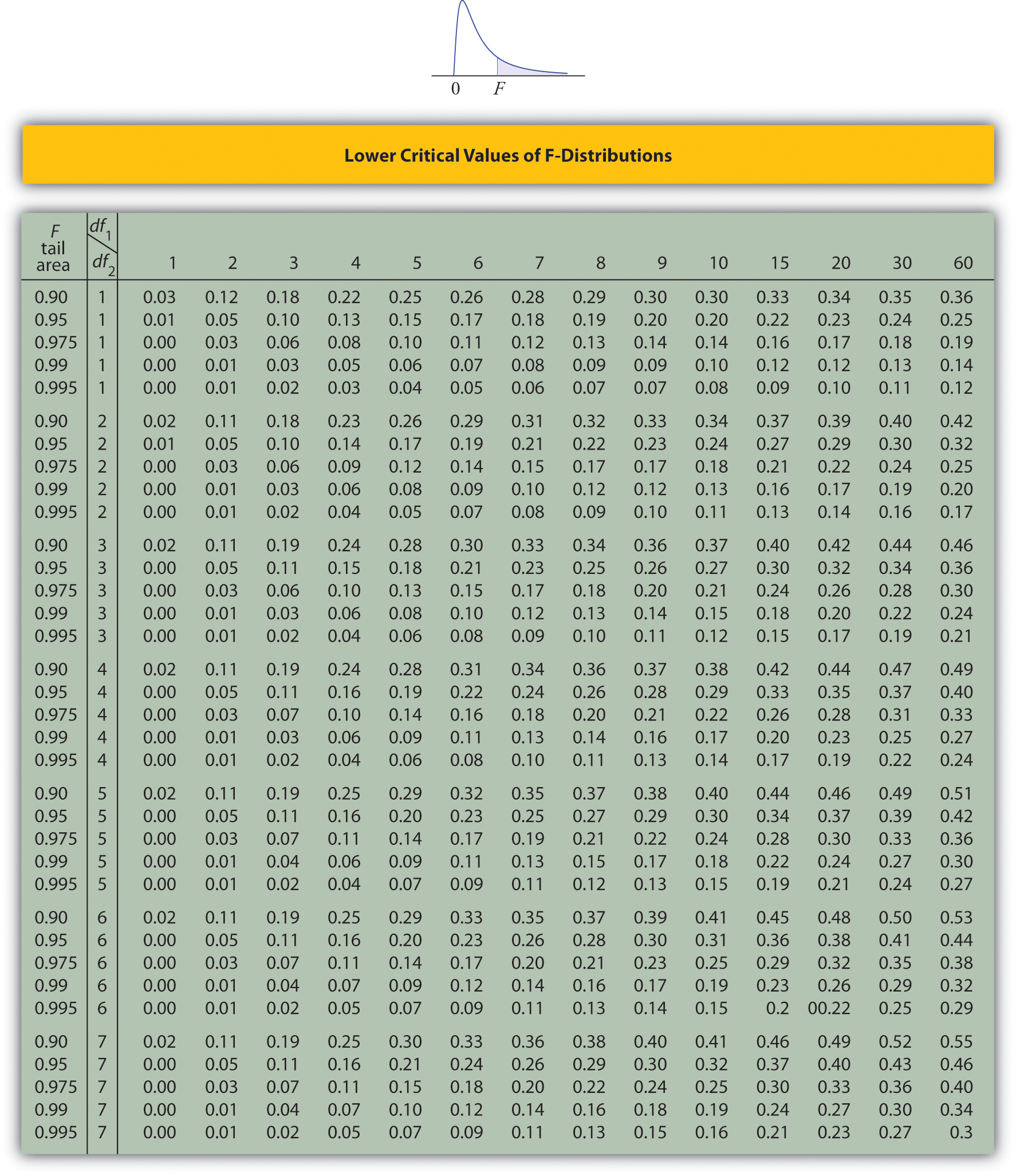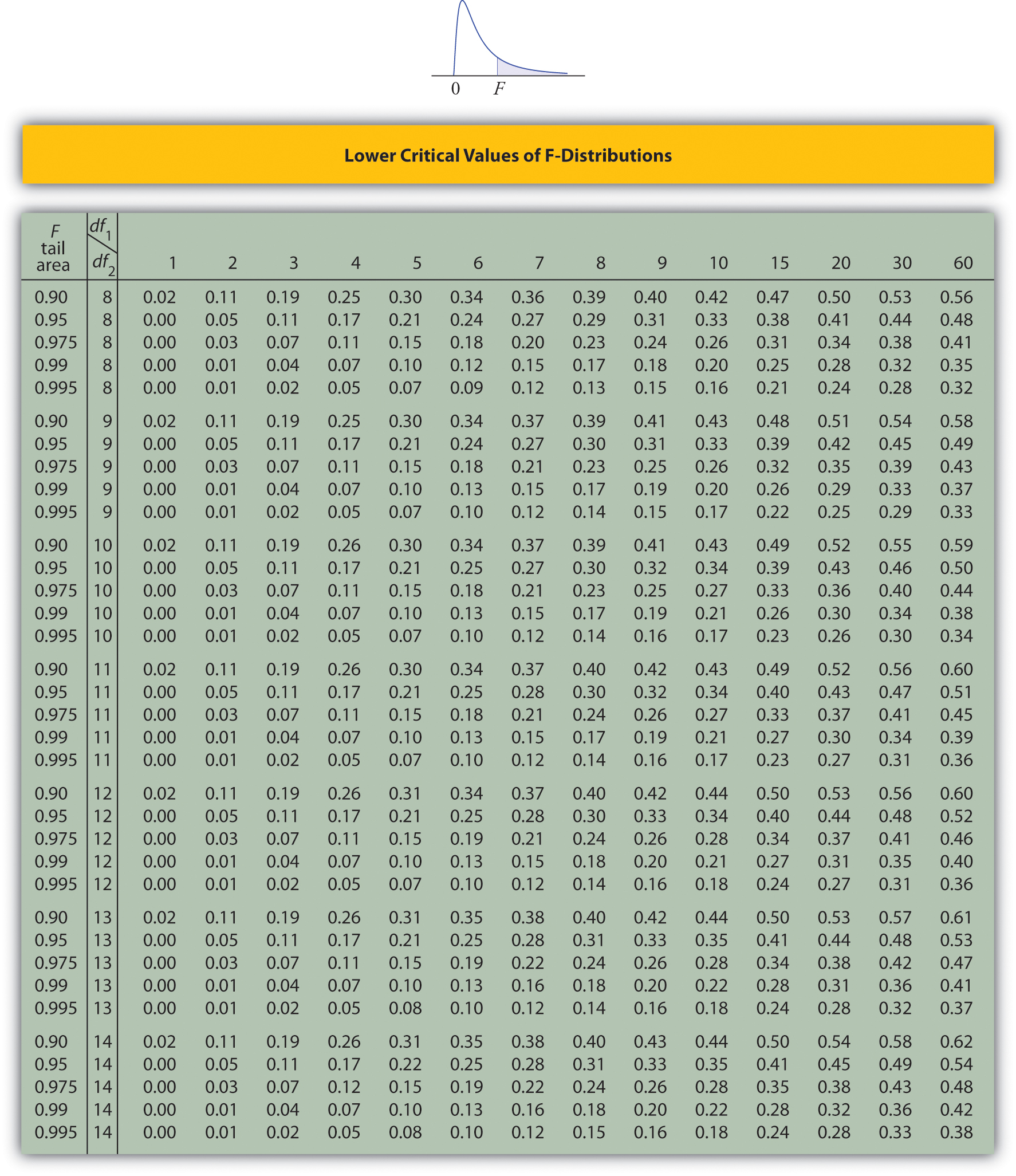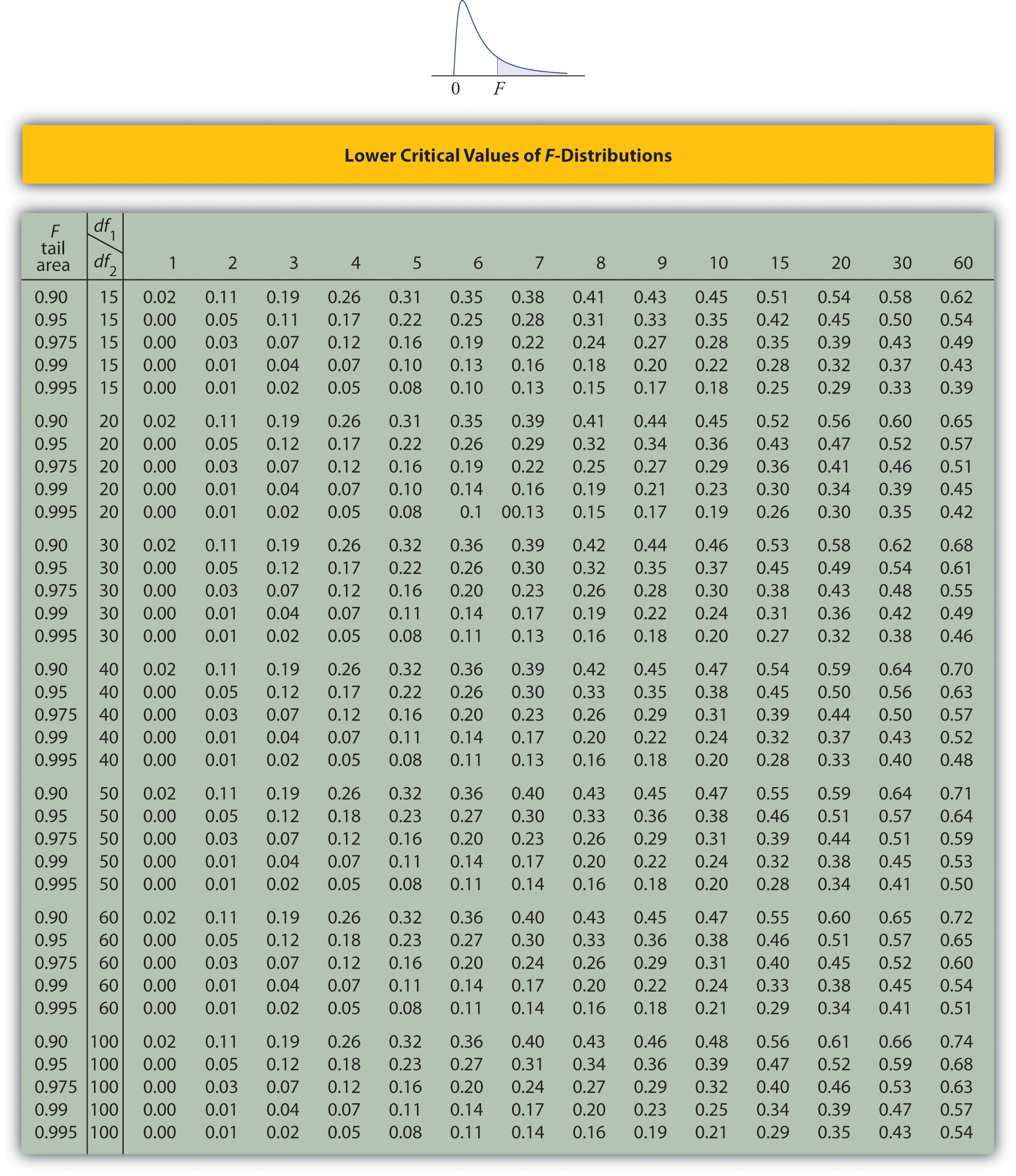# Chapter 12 Appendix

Figure 12.1 Cumulative Binomial Probability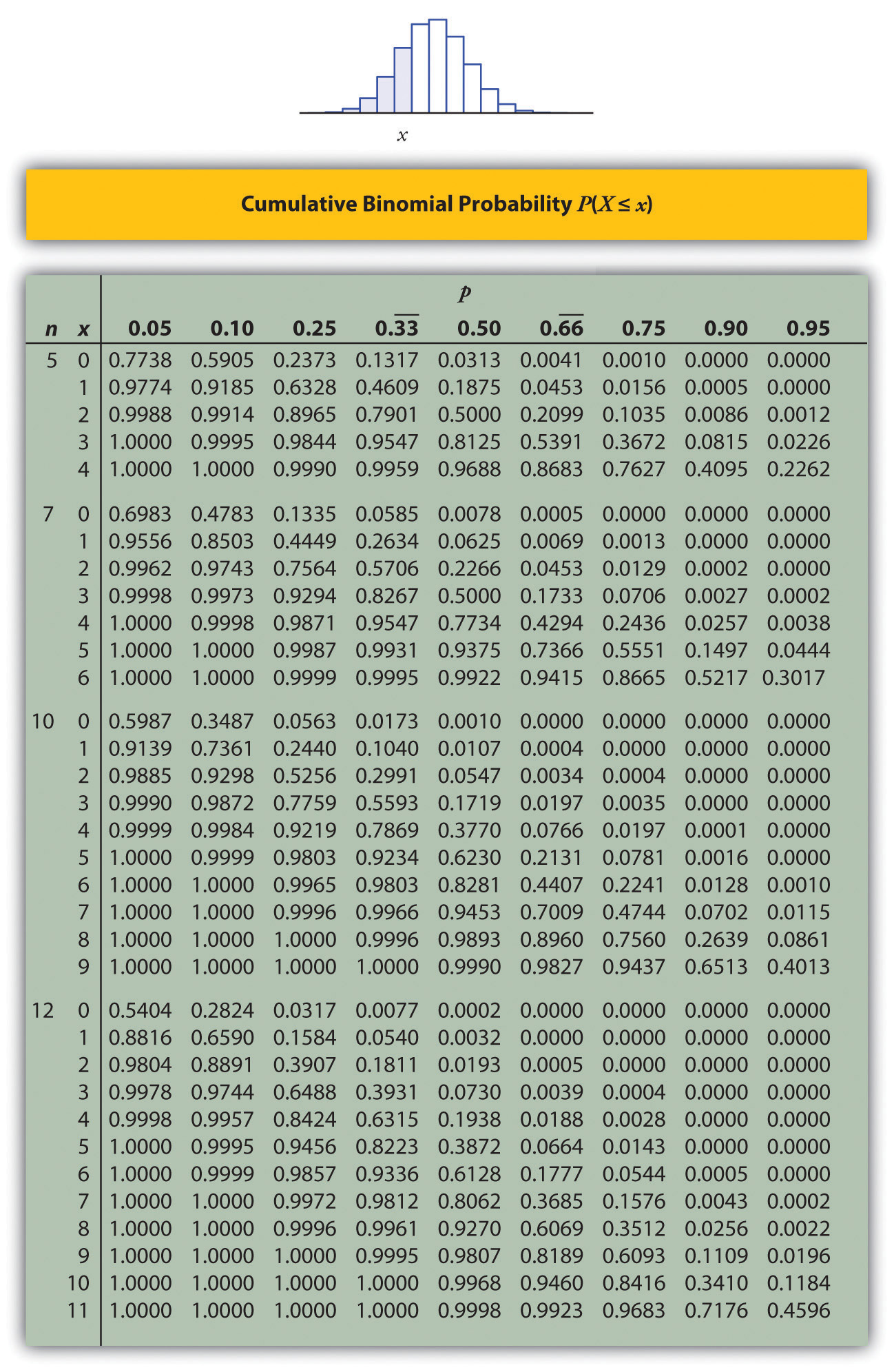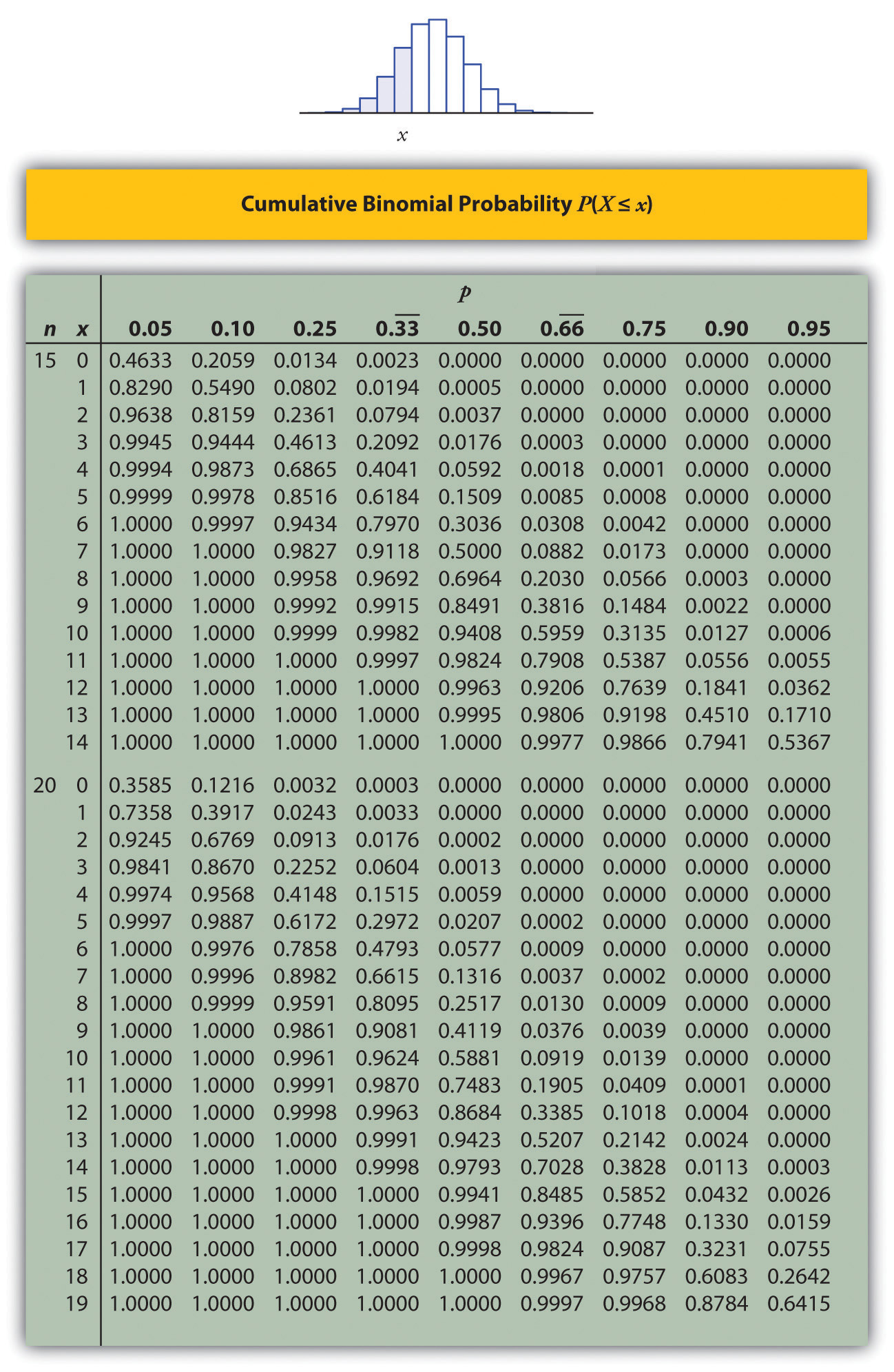Figure 12.2 Cumulative Normal Probability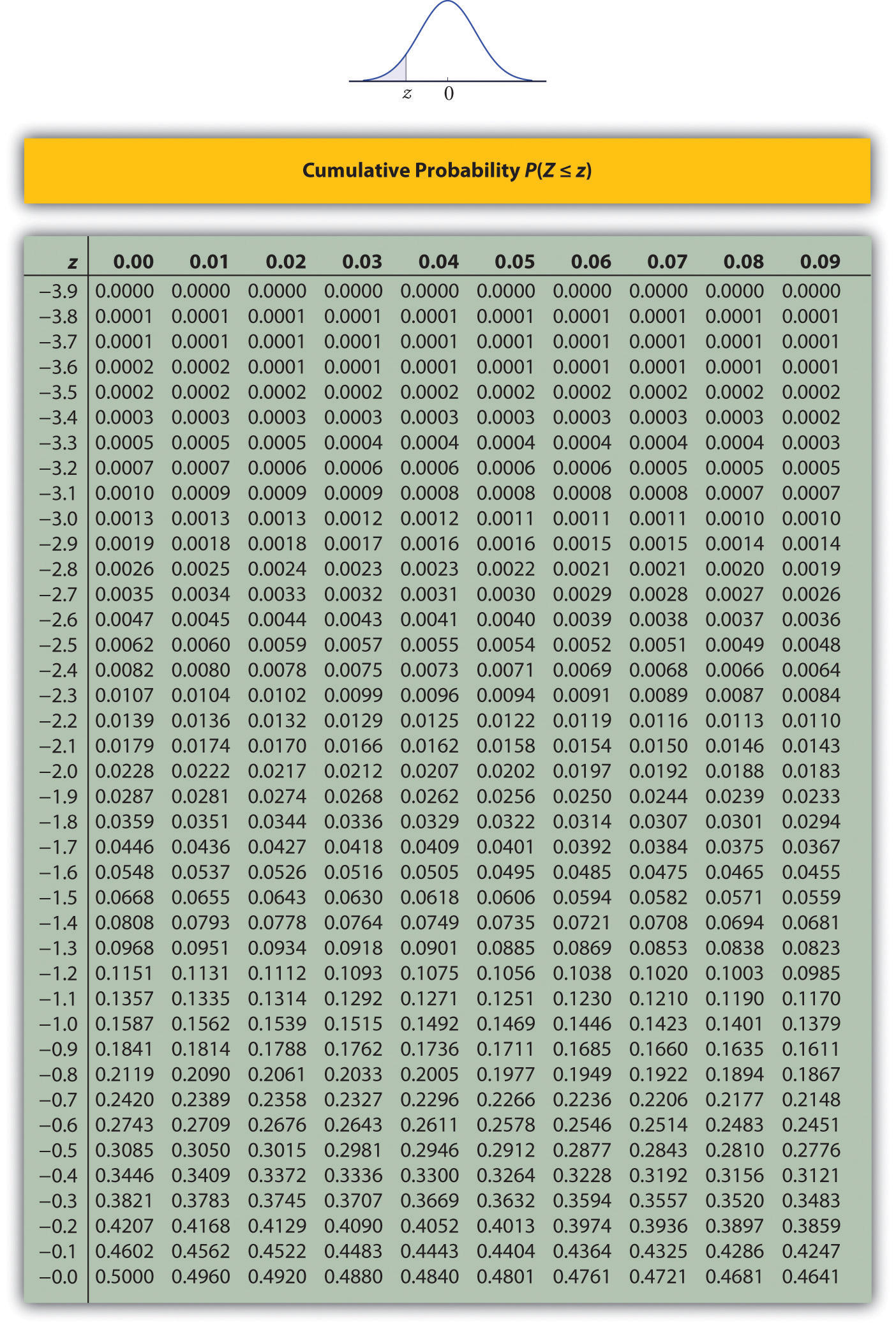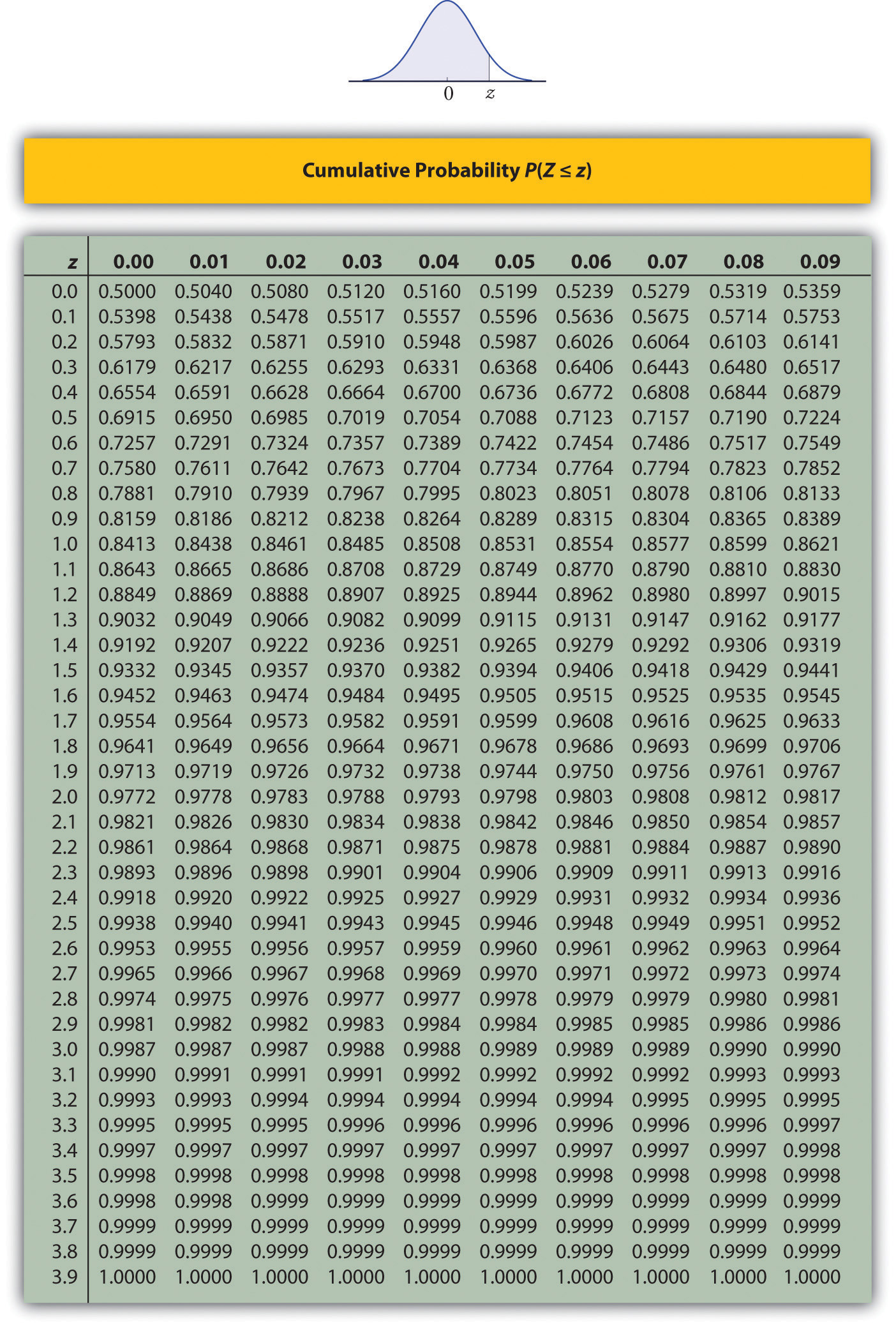Figure 12.3 Critical Values of t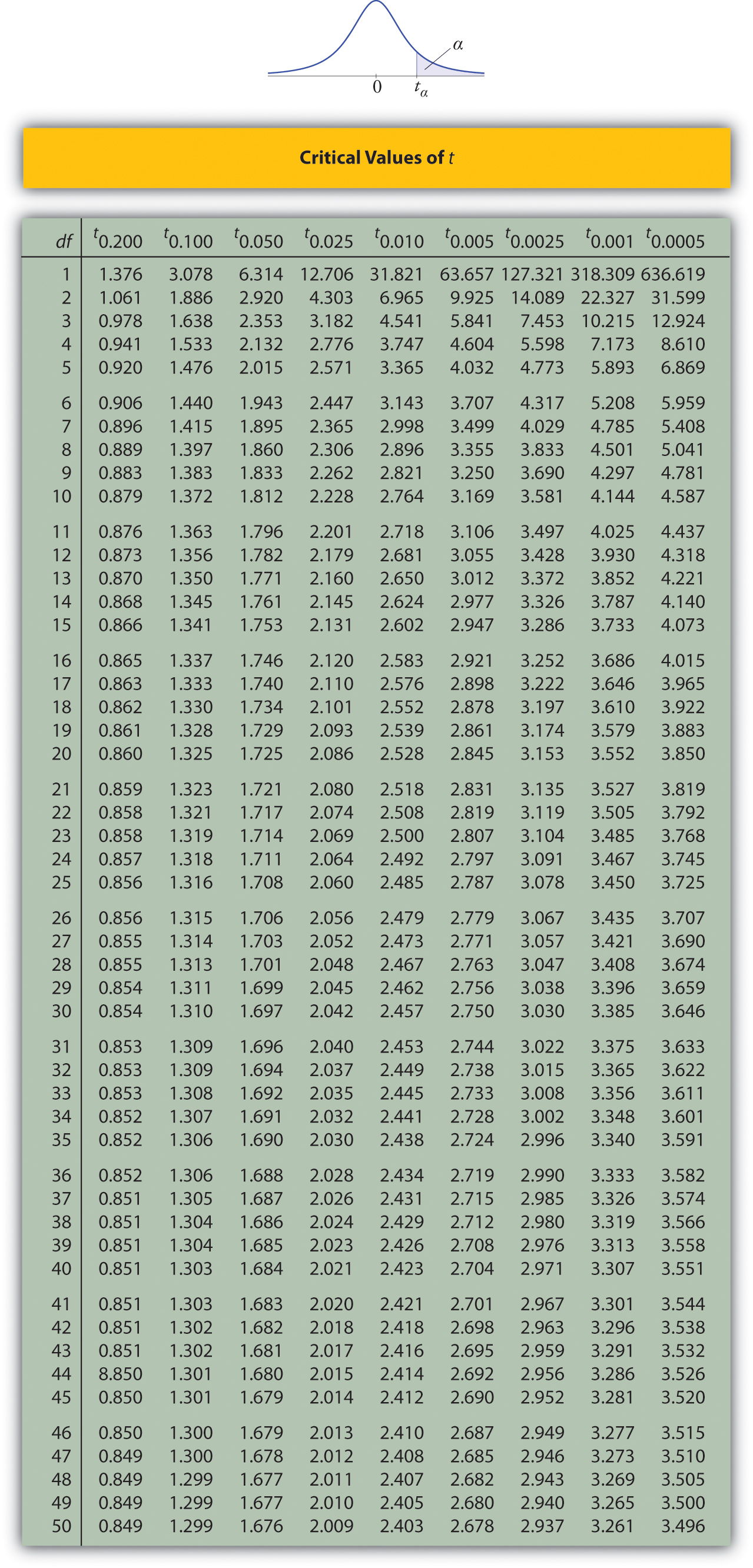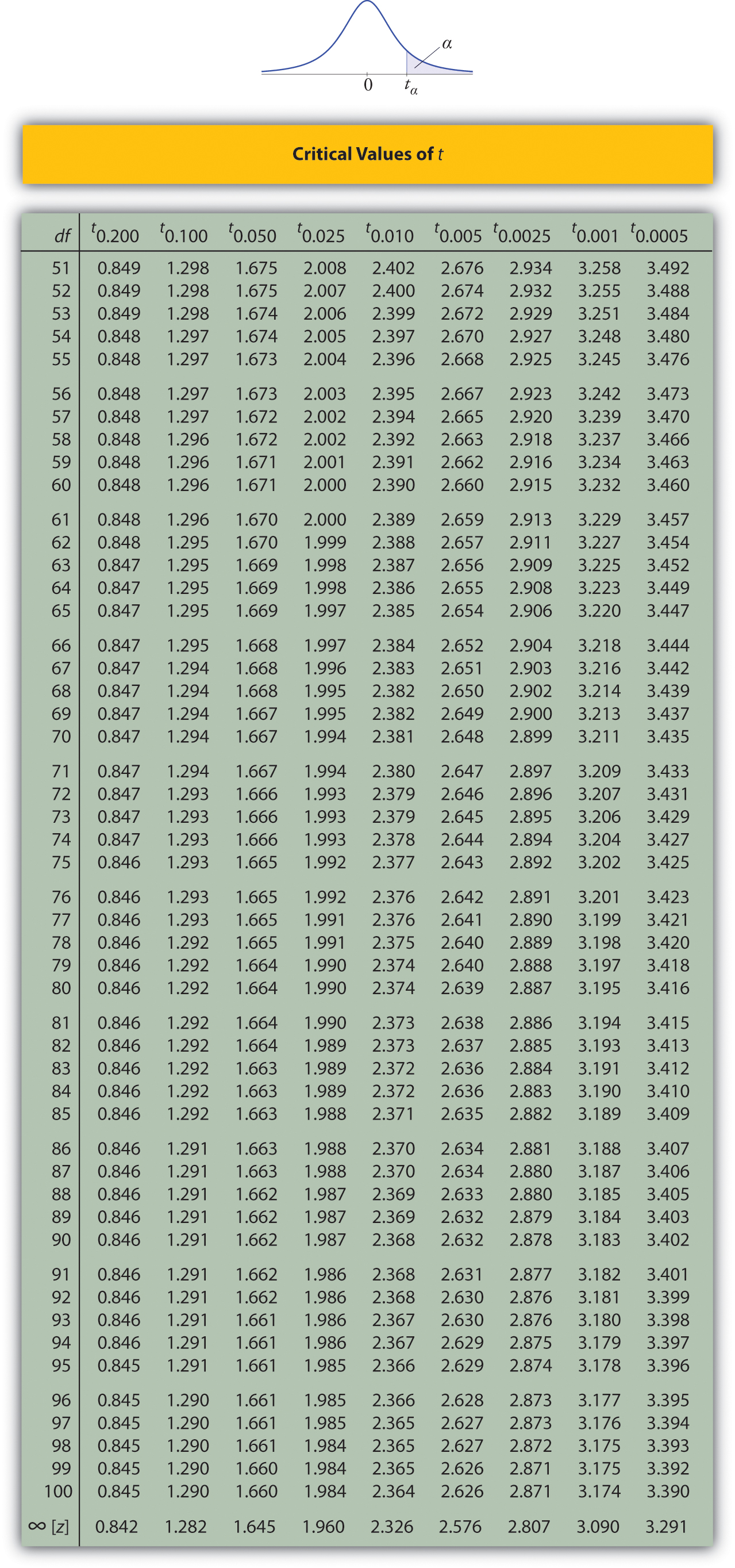Figure 12.4 Critical Values of Chi-Square Distributions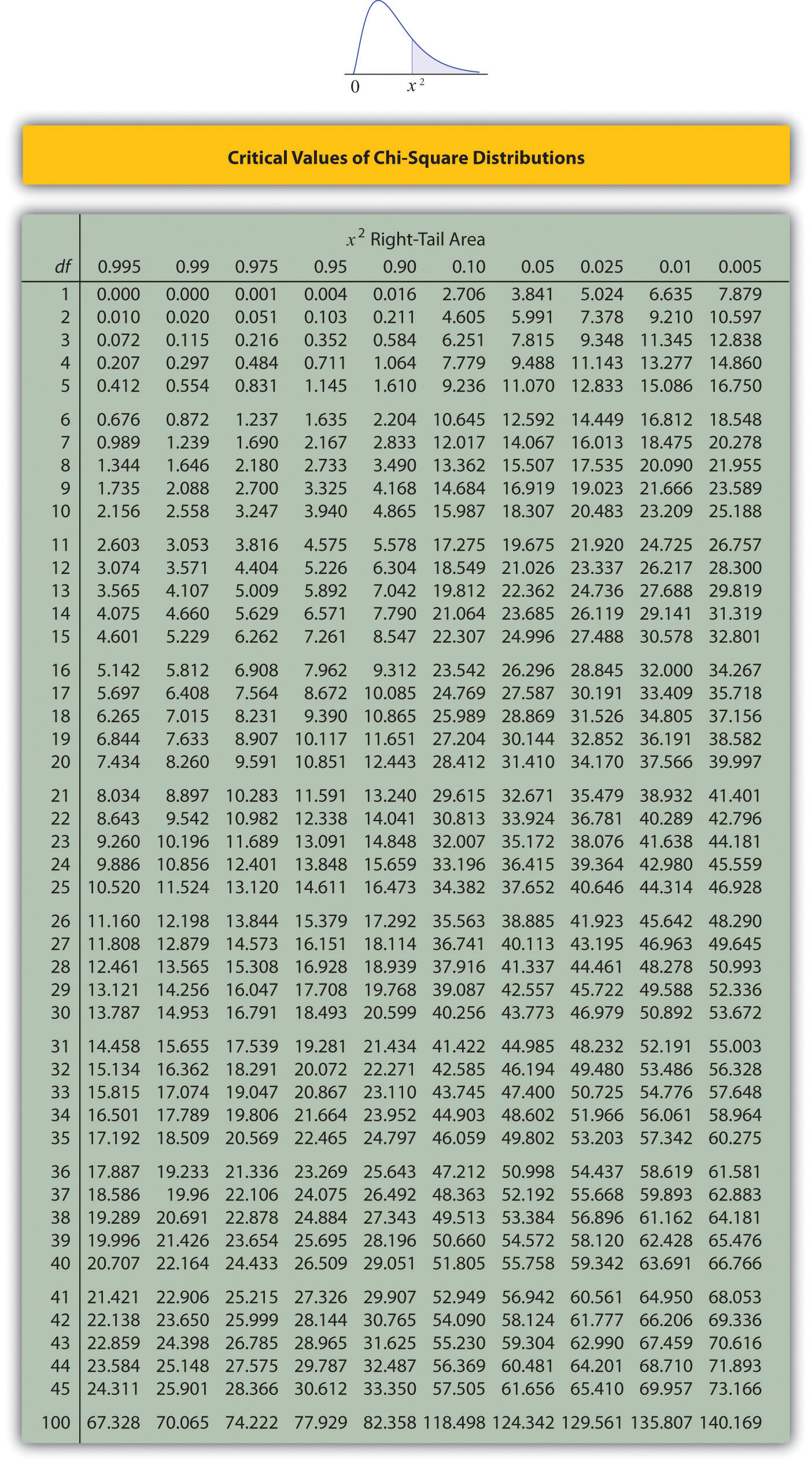Figure 12.5 Upper Critical Values of F-Distributions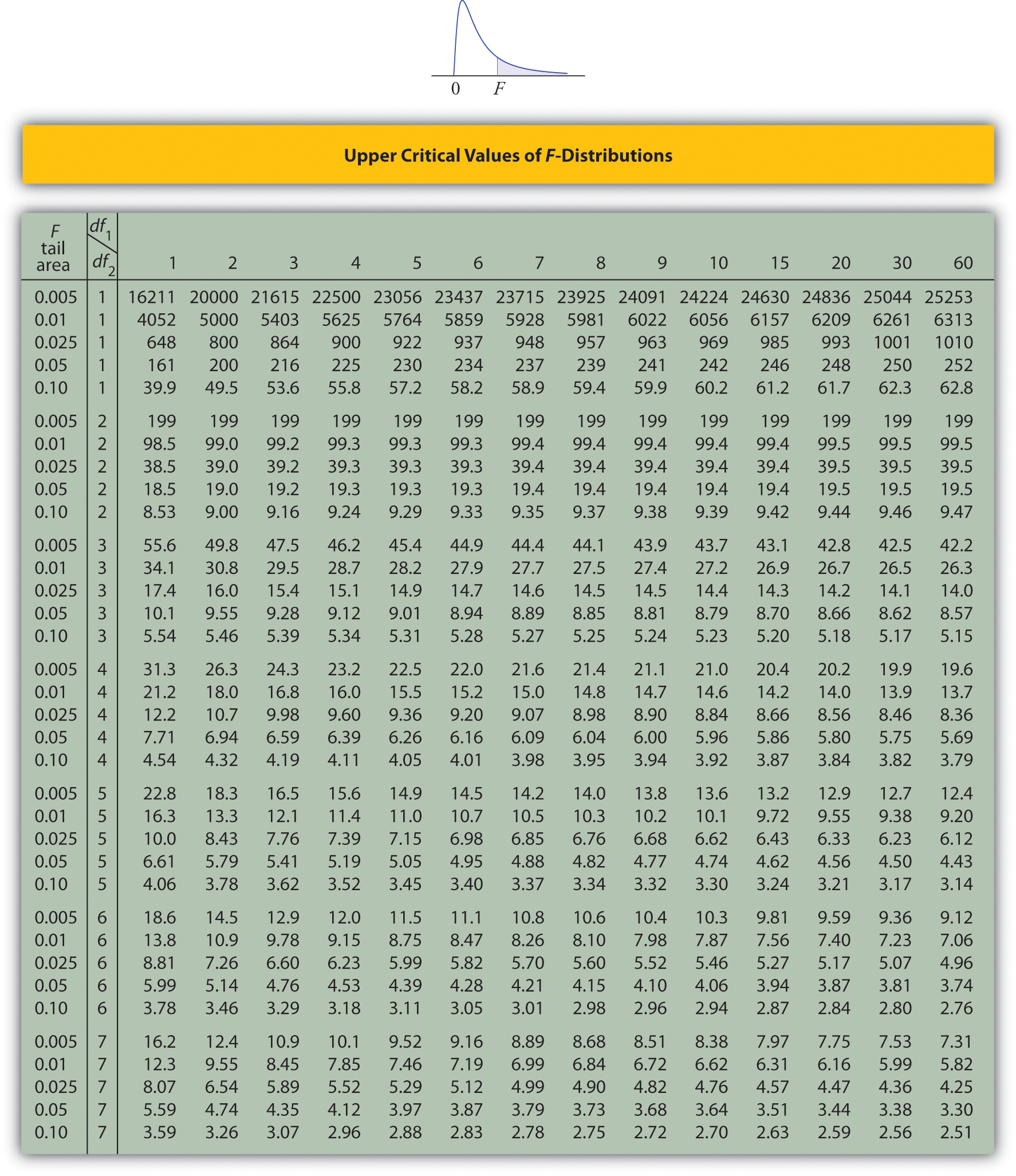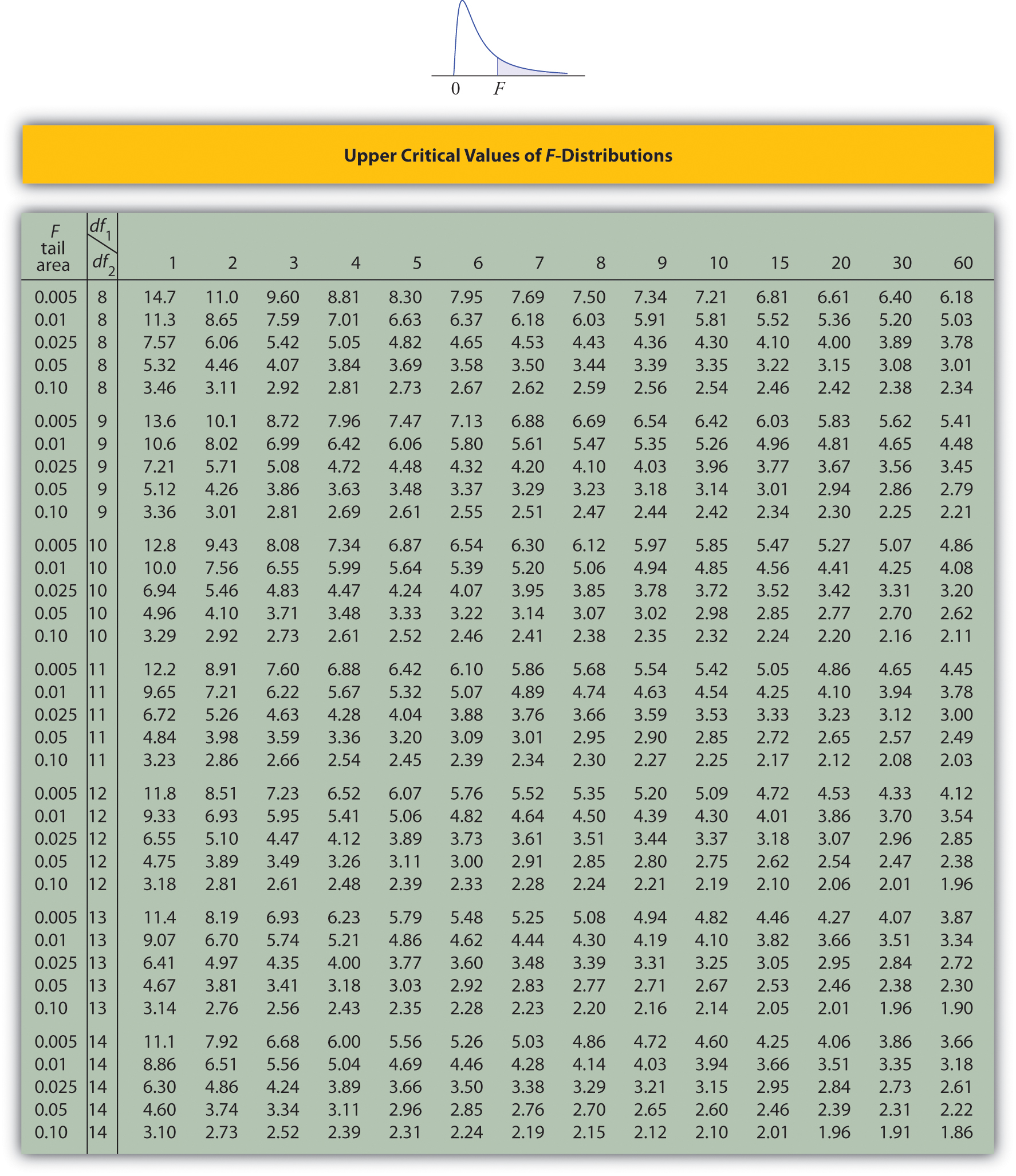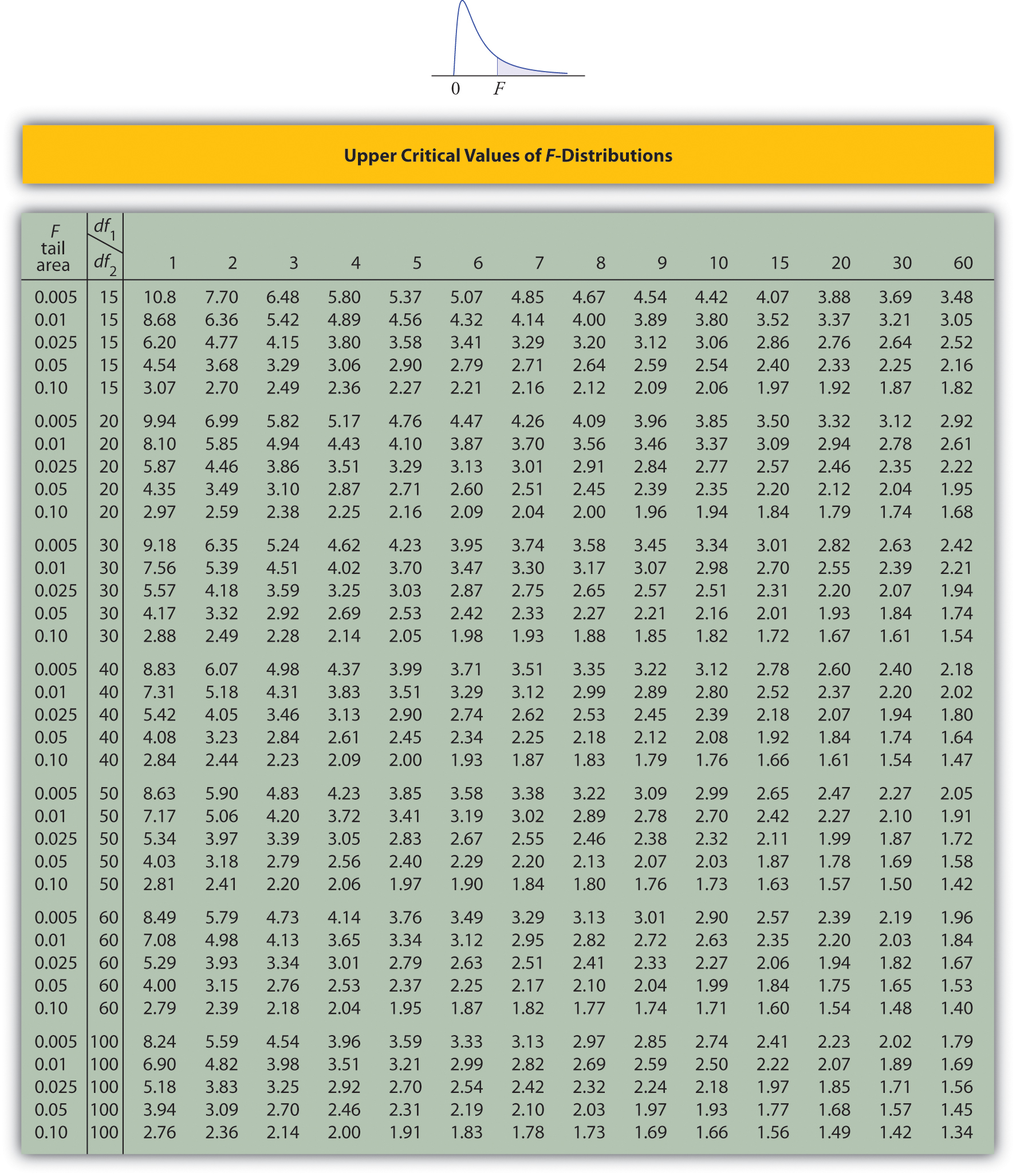Figure 12.6 Lower Critical Values of F-Distributions